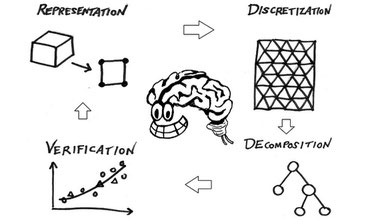# Massachusetts Institute of Technology: Computational Thinking for Modeling and Simulation

Develop the thought processes involved in formulating a problem so a computer can effectively carry out the solution.  In particular, this course emphasizes use of computers for modeling physical systems and predicting their behavior.9 weeks
3–5 hours per week
Instructor-paced
Instructor-led on a course schedule

Computational thinking is becoming widely recognized as a skill necessary for every educated person in a technologically advanced society.

We will focus on just a subset of computational thinking which concerns creating models of the physical world – something that engineers frequently need to do.  Because of that choice, this course covers many topics normally viewed as within the domain of mathematics such as algebra and calculus, but the solution procedures are algorithmic rather than symbolic.

The major themes of the course are:
• Representation -- How do you encode information about the world in a computer?  How do your choices in representation affect the ease with which you can solve problems?
• Decomposition -- How do you break a large and diverse problem into many simpler parts?
• Discretization -- How do you break up space and time into a large number of relatively small pieces?  What are the alternative ways of doing this?  What are the consequences of discretization procedures for accuracy and speed?
• Verification -- How do you build confidence in the results of a model?

### At a glance

• Language: English
• Video Transcript: English
• Associated skills: Forecasting, Calculus, Computational Thinking, Discretization, Algebra

# What you'll learn

Skip What you'll learn
By the end of this course, students will be able to:
• Select and implement methods for interpolation and understand their consequences for convergence of model results as discretization is refined.
• Carry out a few simple methods for numerical integration
• Implement procedures for numerical differentiation
• Write programs to solve systems of equations, both linear and non-linear

# Syllabus

Skip Syllabus
What is Computational Thinking? (representation, discretization, error, decomposition, verification)

Interpolation (building simple surrogates for more complex functions)

Randomness (generating and using pseudorandom variables in models)

Differentiation (numerical derivatives)

Solving equations (Gaussian elimination for linear systems, Newton-Raphson for non-linear systems)